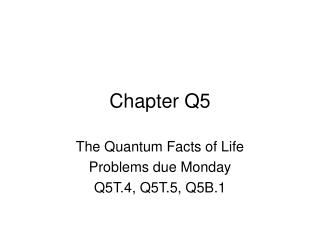DownloadDownload PresentationChapter Q5

# Chapter Q5

Télécharger la présentation## Chapter Q5

- - - - - - - - - - - - - - - - - - - - - - - - - - - E N D - - - - - - - - - - - - - - - - - - - - - - - - - - -
##### Presentation Transcript

1. Chapter Q5 The Quantum Facts of Life Problems due Monday Q5T.4, Q5T.5, Q5B.1

2. What is a “quanton” • Quanton is a name for all forms of matter and energy that exhibit both particle and wave characteristics. • Electrons • Protons • Photons • Neutrons • Atoms • Etc. • The name quanton is not widely used but will be used by our text.

3. Are quantons waves or particles? • Consider the two slit experiment. How can we be sure the pattern we see is caused by wave characteristics and not some interaction between the quantons? By having only one quanton in the apparatus at a time

4. An experiment using a double slit while insuring that only one quanton is present at a time in the apparatus. Laser A series of filters that reduce the number of photons until there is only a 1/1000 chance two photons are present in the apparatus at one time. Bank of detectors, each capable of detecting a single photon. What the detectors see

5. Would this be the result of waves or particles? • Each single result is that of a particle. • The combined statistical result is that of waves. • It is impossible to separate the wave and the particle characteristics of the quantons in this situation • In some situations they may act like particles • Cars • In others they may act like waves. • The experiments you did with light waves. • Why did they act like waves in that situation? • Because there were a very great number of photons present so the statistical result far overrode the single particle effect.

6. More puzzles • How does a particle that goes through one slit know the other slit is there? It can’t be by interaction with other particles, because there is only a 1/1000 chance that another particle will be present.

7. More puzzles • We modify the experiment to include proximity detectors that tell us which slot the quanton goes through without disturbing the particle. • We modify the experiment to include proximity detectors that tell us which slot the quanton goes through without disturbing the particle. • We modify the experiment to include proximity detectors that tell us which slot the quanton goes through without disturbing the particle. • We modify the experiment to include proximity detectors that tell us which slot the quanton goes through without disturbing the particle. What we see before we add the proximity detectors.

8. More puzzles • We modify the experiment to include proximity detectors that tell us which slot the quanton goes through without disturbing the particle. What we see after we add the proximity detectors. The proximity detectors altered the result of the experiment. Their effect was to destroy the wave properties of the quanton.

9. Adding the detectors modified the experiment. • If we know which slot the quanton went through we remove the wave characteristics of the quanton. • It no longer “knows” the other slot is there.

10. Quantons possess a quality called spin. • Spin is a vector quantity, something like angular momentum. • When the spin of a quanton is measured it is always aligned with the axis of the measurement (x, y, or z). • It may have one of two values, + or –, along each axis. • x+, x- • y+, y- • z+, z-

11. The Stern-Gerlach (SG) device • When a stream of quantons enter the SG device they are divided into two streams, one with positive spins, the other with negative spins. • There are three types of these devices, SGx, SGy and SGz. The stream of quantons is divided according to their spins in the x direction + SGx Quantons with positive spins exit here in - Quantons with negative spins exit here Stream of quantons enter here

12. We can do Stern-Gerlach experiments with real particles and photons. Below are some of the results 100% + SGx in 50% + SGx in - - 50% When a beam of quantons with a random mixture of spins enters the SG apparatus, the quantons are separated into two equal beams, one with spin up, the other spin down. If the beam entering the SG apparatus has spins aligned, they will all come through in the same beam. + SGx 50% in + SGy 50% in - 50% + SGx 50% in - 50% - If the spins are aligned in the x direction then separated in the y direction, the y direction alignment destroys the x alignment.

13. More Stern-Gerlach experiments + + SGz SGx 100% in in + SGx in - - - When we recombine the two outputs so we no longer know the spin in the z direction, we regain full knowledge of the spins in the x direction.

14. Complex numbers • We will need these in doing quantum mechanics where You may put the complex number rules from pages 89-90 on your formula sheet. Of special interest is the complex conjugate (c*).

15. Wave functions in quantum mechanics The inner product of two vectors is defined as: Two vectors are orthogonal if their inner product is zero. A vector is normalized if its magnitude is one.

16. Problems due Monday • Q5T.4, Q5T.5, Q5B.1